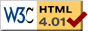### Journée-séminaire de combinatoire

#### (équipe CALIN du LIPN, université Paris-Nord, Villetaneuse)

Le 16 novembre 2021 à 14h00 en B107 (& visioconférence), Julien David nous parlera de : Une nouvelle approche pour l'analyse d'algorithme en moyenne: le cas de l'entropie de Shannon

Résumé : The average-case analysis of algorithm is often an object of controversy. Some would say that it is not a relevant information of the algorithm’s "true" efficiency as it is often based on the uniform distribution over the inputs, which is not a good modelization of real-world contexts. Others would reply that the same can be said about worst-case analysis and that, in this regard, average case analysis is a non negligible additional information when one has to choose between two algorithms to solve a problem. In this presentation, we adopt the following point of view: the average case analysis of an algorithm is relevant to predict the algorithm behavior in a real-world context if and only if there exists a property (either on the distribution or the object), determining for the algorithm efficiency, whose average value is approximately the same given the random distribution under which the algorithm is studied and in the real-world context. In this study, the "determining property" we consider is the Shannon entropy of probability distributions. We exhibit several random generators of "probability distributions with fixed entropy" and perform an experimental and theoretical analysis of string matching algorithms. We obtain a continuous function, which takes a Shannon entropy as an input and returns the average complexity of the naive string matching algorithm. Joint work with Olivier Bodini and Izabell Iskandar.

[Slides.pdf] [vidéo]

 Dernière modification : Monday 24 January 2022Contact pour cette page : Cyril.Banderier at lipn.univ-paris13.fr